﻿ Lab Work on Electronics Официальный сайт УНЦ ОИЯИ

### Lab Work on Electronics

The Lab Work on Electronics is aimed at hands-on acquaintance with the main radio-electronic components. Unlike other engineering lab works, this one includes minimum equipment requirements: a signal generator, an oscilloscope, a tester, and a soldering iron as the main tool. Students do not work with specially designed training models, but with real radio components: resistors, capacitors, diodes, transistors, etc. The Lab Work begins with an introduction to the main elements — resistance / capacitance / inductance. Afterwards, filters, semiconductors (diodes, transistors) are studied. The basic course ends with assembling a simple preamplifier for a particle detector. Advanced course covers more complicated amplifiers and time-to-time converter.

Prerequisites:

• basics of mathematical analysis, linear algebra and analytic geometry
• basics of physics: metric prefixes, basics of electricity
• basics of computer knowledge: MS Windows, MS Office
• appropriate glasses/lenses if the student has poor eyesight: the training includes work with small elements

Before starting the Lab Work, students are required to do the safety (work with a soldering iron, bare low-current live conductors, fluxes and solder) and fire safety training.

### List of Lab Works (B — basic course, A — advanced course):

 1. (B) Introduction to the equipment. Soldering basics Result: skill of work with a multimeter, an oscilloscope, a signal generator and a soldering iron.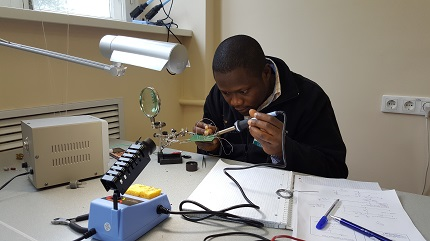2. (B) Introduction to the linear electric circuit components Result: skill of calculation of the branched passive component circuits (calculation of currents and voltages at different circuit sections; calculation of dissipation power and the resulting choice of elements).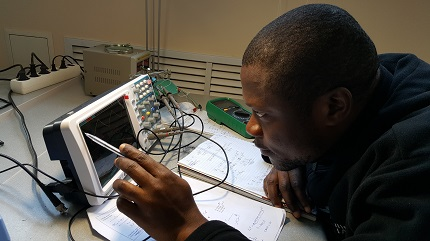3. (B) Calculation of parameters for high-, mid- and low-pass filters Result: skill of calculating and constructing the frequency filter with required parameters (cut-off frequency, load, amplitude).4. (B) Study of semiconductor properties Result: hands-on skill of work with diodes: general idea of the p-n-junction, diode test using a multimeter, cathode and anode finding, diode applications (signal amplitude limitation, reverse current liquidation, diode bridge etc.)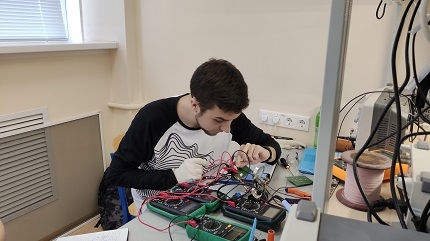5. (B) Power amplifier based on the common emitter (CE) bipolar junction transistor (BJT) topology Result: general idea of the BJT and its operation modes. CE BJT electronic relay circuit: practice of calculating, choosing components, circuit assembling and testing. Transition from the electronic relay circuit to the power amplifier circuit: skill of calculating and constructing the amplifier with the required voltage gain.6. (B) Power amplifier based on the common collector (CC) BJT topology Result: practice of calculating, choosing components, circuit assembling and testing the CC power amplifier with the required parameters (current gain, input / output resistance). Skill of combining several amplifiers (CE and CC) and evaluating the characteristics of each topology.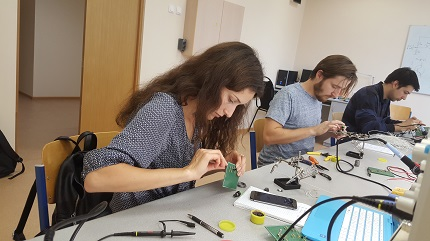7. (B) Power amplifier based on the common base (CB) BJT topology. Construction of the preamplifier for a cosmic ray detector Result: practice of calculating, choosing components, circuit assembling, and testing the voltage stabilizer for a specific task, as well as the CB power amplifier. Skill of combining CB and CC circuits to the preamplifier for a cosmic ray detector. Understanding the current distribution in the preamplifier circuit. Skill of working with real equipment: debugging and testing the charge-sensitive amplifier circuit with a real cosmic ray detector.8. (A) Charge-sensitive amplifier on bipolar transistors for a cosmic rays detector Result: skill of calculating the differential amplifier (DA), understanding of the direct and signal currents in the DA. Skill of calculating and constructing the charge-sensitive amplifier based on DA, CE and CC. Skill of working with the real equipment: charge-sensitive amplifier circuit debugging and testing with the real cosmic rays detector.9. (A) Operational amplifiers and feedbacks: from discrete components to integral circuits Result: general idea of the operational amplifiers (OA) and their parameters, general idea of the feedbacks (FB). Skill of visual defining the FB type at the circuit, ability to choose the convinient FB type for a certain task. Skill of calculating and constructing the amplifier based on OA (LM 358 P precision OA is used in the Lab Work).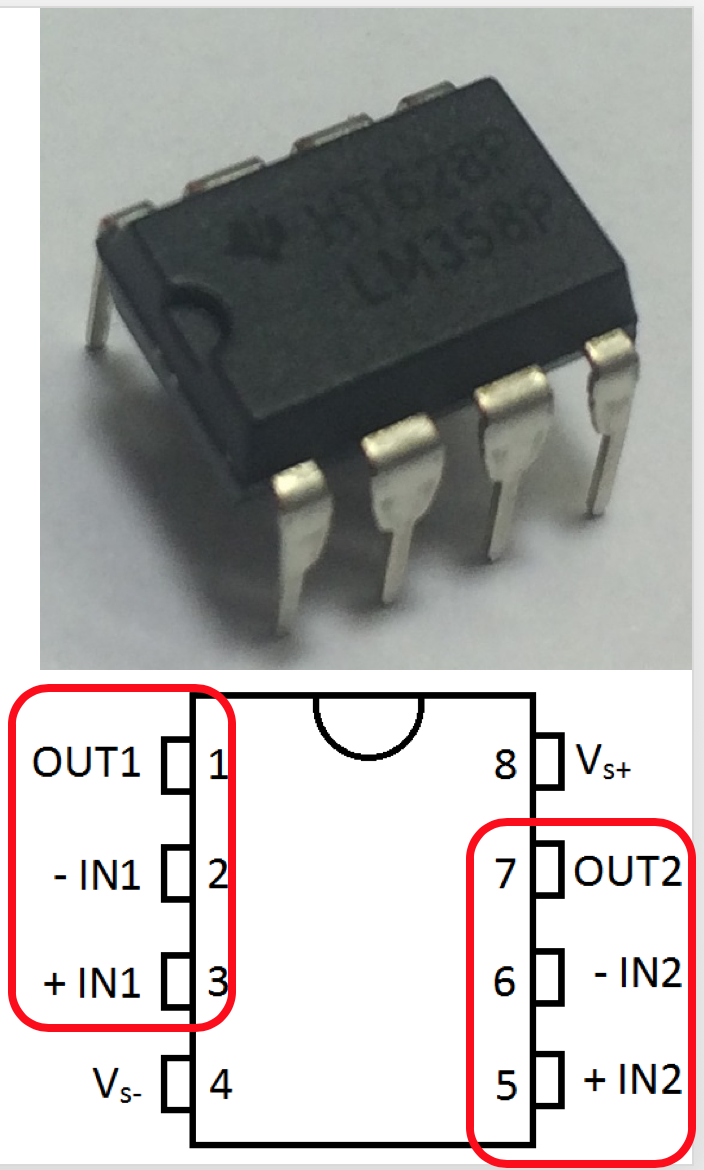10. (A) Investigation of the dependence of the delay time on the cable length using the time-to-time converter Result: general idea of the current mirror, direct and differential currents in it, calculation and application. Skill of calculating and constructing of the time-to-time converter (TTC) on transistor keys. Ability to evaluate TTC transformation ratio.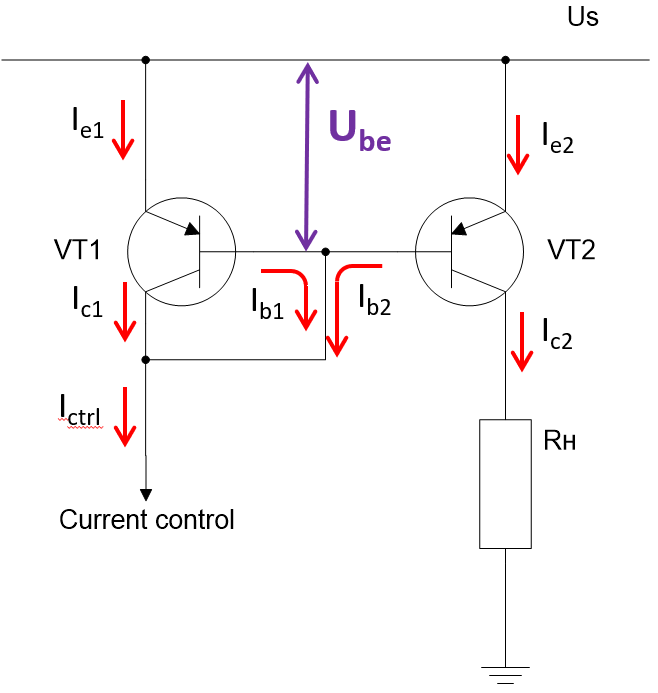11. (A) Transient processes and relaxation devices Result: general idea of the transient processes in carious RC circuits. Skill of calculating and constructing the multivibrator with RC circuits with required frequencies. General idea of the triggers (SR latch, Shmitt trigger) and their application.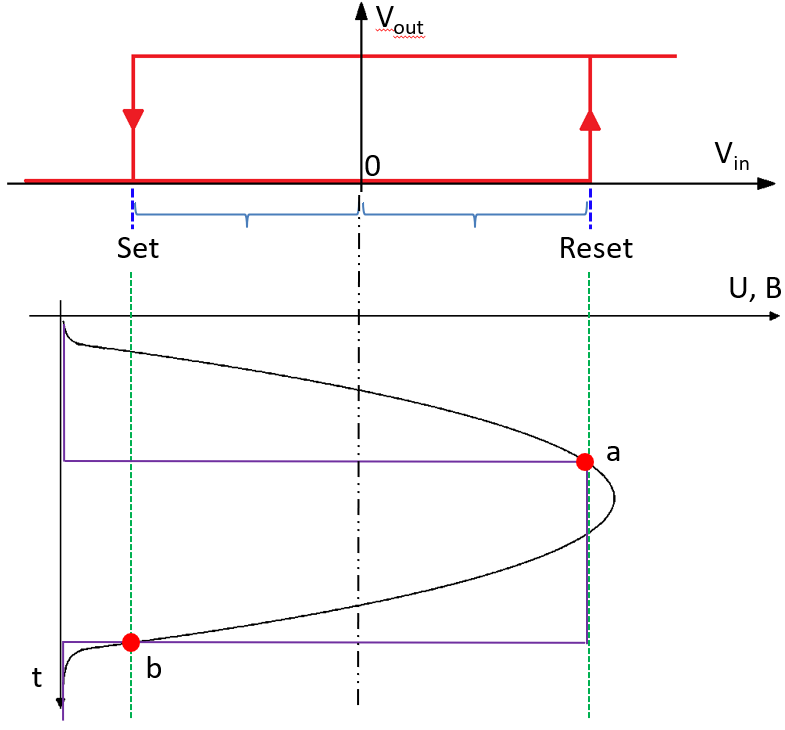Full course is designed for 3 weeks. However, lab works duration highly depends on student's level and basic skills. Depending on that the amount of the lab works in the course may vary. It is also possible to shorten the course by removing some lab works.